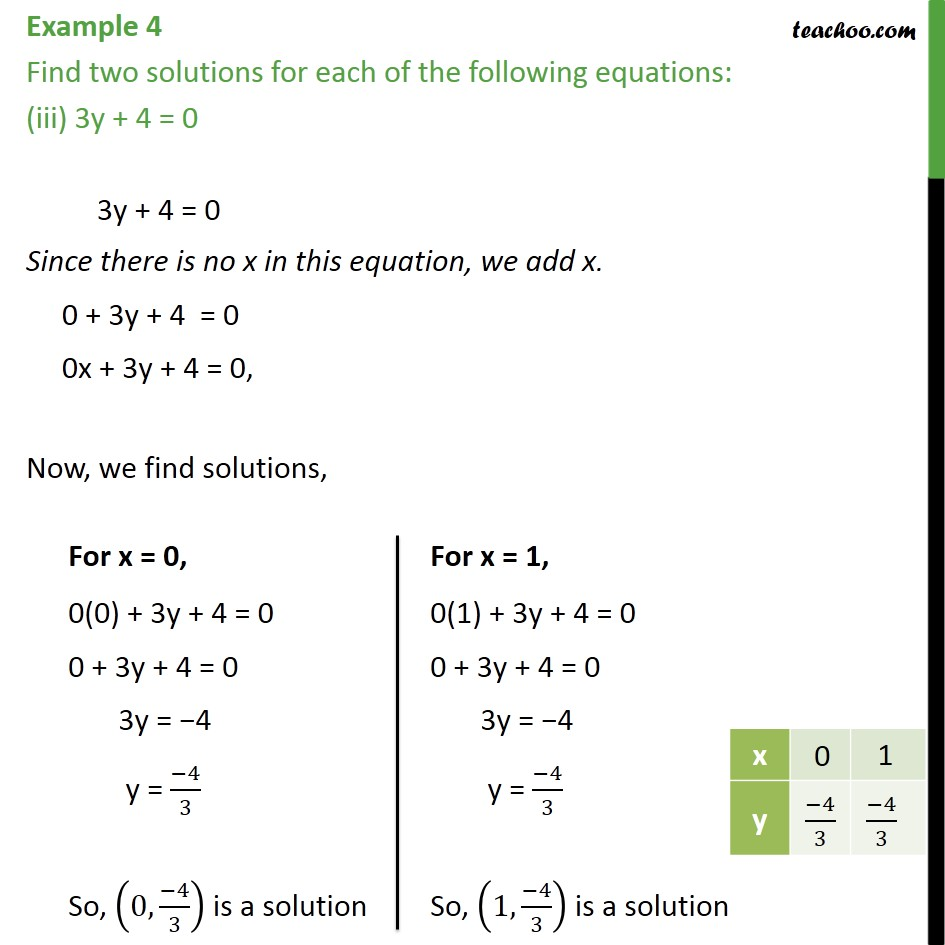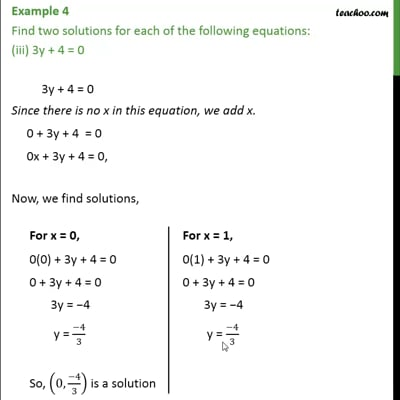Examples

Chapter 4 Class 9 Linear Equations in Two Variables
Serial order wiseThis video is only available for Teachoo black users

Introducing your new favourite teacher - Teachoo Black, at only ₹83 per month

### Transcript

Example 4 Find two solutions for each of the following equations: (iii) 3y + 4 = 0 3y + 4 = 0 Since there is no x in this equation, we add x. 0 + 3y + 4 = 0 0x + 3y + 4 = 0, Now, we find solutions,